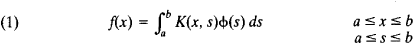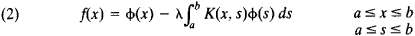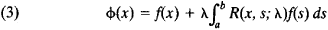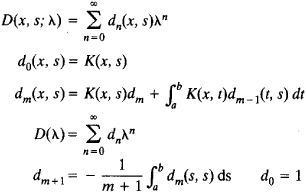# Fredholm Equation

The following article is from The Great Soviet Encyclopedia (1979). It might be outdated or ideologically biased.

## Fredholm Equation

The Fredholm equation of the first kind isThe Fredholm equation of the second kind isHere, K(x, s) is a given continuous function of x and s called the kernel of the equation, f(x) is a given function, ϕ(x) is the unknown function, and λ. is a parameter (seeINTEGRAL EQUATIONS).

Equations (1) and (2) were studied between 1900 and 1903 by E. Fredholm. The theory of Fredholm equations of the second kind is simpler, and these equations are more often used in applications. In the general case it is possible to obtain stable solutions of Fredholm equations of the first kind only with the help of special regularizing algorithms for the solution of incorrectly posed problems.

If λ is not an eigenvalue of equation (2), the equation has a unique continuous solution defined by the formulawhere, R(x, s; λ) = D(x, s; λ)/D(λ) is called the resolvent of equation (2). Here,